Optimal Design Of Risk Control Strategy Indexes

August 17, 2012

Appendix 5: Optimal Target Volatility Indices

As a next step we are looking for an optimal target volatility response function, i.e. we are
looking for the response function that maximizes the expected return for a given level of expected volatility T: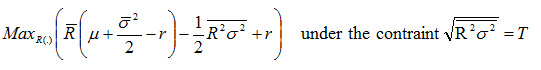(23)

In contrast to the target volatility strategy discussed above, the optimal risk strategy only targets a certain volatility level T on average, whereas the risk control function (6) is designed to keep the investment strategy at the desired volatility level at all times.

To optimize the expected return for a given level of expected volatility we can plug the constraint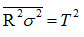(24)

into the expected return (12) and at the same time take into account this constraint via a Lagrange-parameter l in the following Lagrange-function: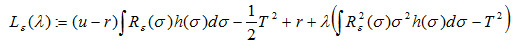The first order optimality condition reads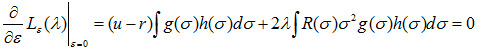Substituting g(.) for the delta distribution ?(?-?') and renaming ?' back to ? yields: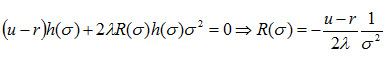(25)

To determine ? we use (25) in the constraint (24):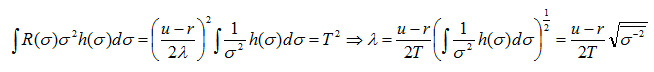With which the risk-return optimal response function (25) reads: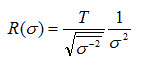(26)

The expected return of the optimal risk strategy index reads: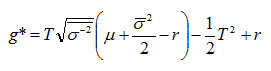(27)

The Sharpe-ratio becomes: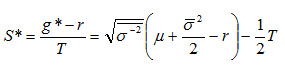(28)

The Sharpe-ratio (28) is always greater than the Sharpe-ratio of the underlying index and greater than the Sharpe-ratio of the target volatility index (18) because in general we have: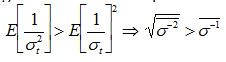This is a particularly strong result since it shows that the Sharpe-ratio of the target volatility index (which already improves the Sharpe-ratio of the underlying equity index as shown above) is even further improved, making it an ideal underlying for financial products that are designed to offer equity returns with the best possible risk controlling mechanism to investors. As for the target volatility scheme, the improvement in Sharpe-ratio compared to the underlying index is driven by the volatility of the volatility of the underlying index, since in the expected return (27) we have in second order approximation: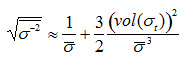It is interesting to compare the response function of the risk control strategy (6) to the optimal risk response function (26). The target volatility strategy is designed to keep the volatility of the investment scheme constantly at the target level T. However, the optimal risk response function (26) only targets the volatility level T on average and shows a stronger response to volatility in the sense that in equity markets with relatively low levels of volatility the equity investment is geared up such that investment portfolio is above average volatile. On the other hand, when equity volatility is relatively high the equity investment is reduced such that the investment portfolio is below average volatile. To conclude, instead of keeping the volatility of the portfolio at a constant level the optimal risk strategy invests counter-cyclically, i.e., it takes more risk when equity markets are in a regime of low volatility but takes less risk when markets are above average volatile.